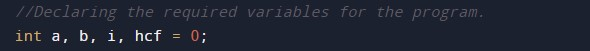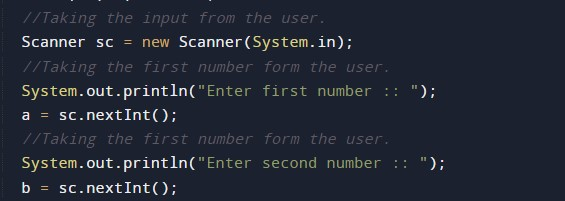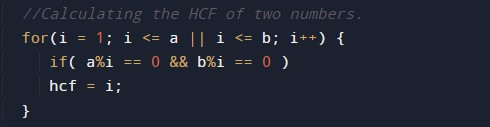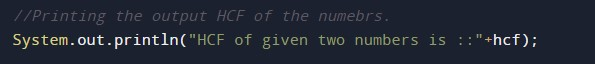# Java Program to Find HCF of two numbers

In this tutorial you will learn about the Java Program to Find HCF of two numbers and its application with practical example.

## Java Program to Find HCF of two numbers

In this tutorial, we will learn to create a Java program that will find the HCF of two numbers using Java programming.

## Prerequisites

Before starting with this tutorial we assume that you are best aware of the following Java programming topics:

• Operators in Java Programming.
• Basic Input and Output function in Java Programming.
• Basic Java programming.
• While loop in Java programming.
• Arithmetic operations in Java Programming.

## Program Description:-

In Java programming, it is possible to take numerical input from the user and find HCF of two numbers with the help of a very small amount of code. The Java programming language has many types of predefined libraries which have support for the function in them with the help of these arithmetic expressions we can find the HCF. The program first takes the two numbers in input then it will find the HCF using the for loop and the conditional statements. At last, we will print the output HCF to the user.

With the help of this program, we can find HCF.

Here,

HCF:- Highest Common Factor.

## Output:-In the above program, we have first initialized the required variable.• a = it will hold the integer value1.
• b = it will hold the integer value2.
• t = it will hold the integer temporary value.
• hcf = it will hold the integer value of calculated hcf.

Input number from the user.Program Logic Code.Printing outputIn this tutorial we have learn about the Java Program to Find HCF of two numbers and its application with practical example. I hope you will like this tutorial.